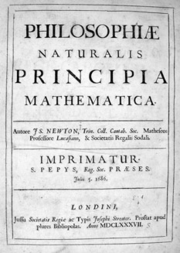# On this day July 5, 1687

The Philosophiae Naturalis Principia Mathematica by Isaac Newton was first published, describing his laws of motion and his law of universal gravitation.The Principia is a three-volume work, Newton also published two further editions, the second in 1713, and the third in 1726.

The Principia contains the statement of Newton’s laws of motion forming the foundation of classical mechanics, as well as his law of universal gravitation and a derivation of Kepler’s laws for the motion of the planets (which were first obtained empirically).

The Principia is “justly regarded as one of the most important works in the history of science”.

In formulating his physical theories, Newton had developed a field of mathematics now known as calculus. However, the language of calculus as we know it was largely absent from the Principia. Instead, Newton cast the majority of his proofs in geometric form, although with many calculus-like arguments based on limits of vanishing small geometric quantities.

Tags: ,